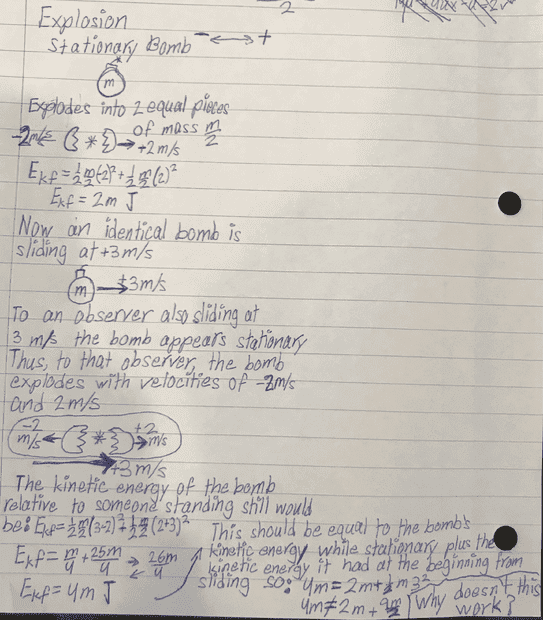# Conservation of Energy and Momentum in an Explosion

• B
• JamesG23
In summary, the kinetic energy of the bomb pieces after explosion is the same whether they are moving or stationary.f

#### JamesG23

Hey, I have a question about explosions and how kinetic energy works during them. I have outlined my question on the attached image. Please let me know if something is wrong or needs clarifying. Thank you.•berkeman
It would be easier to comment if you had typed up your work. But anyway:

Viewed from the lab frame, you calculated the total KE after the explosion = (26m)/4. Sounds good. Not sure why you set that equal to 4m.

The KE before explosion = (1/2)m(9) = (18m)/4. Subtract that from the KE after the explosion and see what you get.

It would be easier to comment if you had typed up your work. But anyway:

Viewed from the lab frame, you calculated the total KE after the explosion = (26m)/4. Sounds good. Not sure why you set that equal to 4m.

The KE before explosion = (1/2)m(9) = (18m)/4. Subtract that from the KE after the explosion and see what you get.
Oh shoot I don't know why I simplified like that. Maybe I thought it was 24/6. Thank you

I have a question about explosions and how kinetic energy works during them.
In an atmosphere, the explosion of a flying bomb produces a sphere of hot combustion gas that has a very low density compared to the original explosive charge.
That sphere is effectively stopped immediately by it's low mass and the area of it's greater cross-section.
The original KE is not lost, it is just insignificant when applied to the huge mass of atmosphere that encloses the explosion.

Let's say the bomb pieces are 10kg each.
KE of each piece at ±2 m/s: 1/2mv2=20J
Total KE of bomb pieces: 40 J

KE of both bomb pieces at +3 m/s before detonation: 90 J
KE of bomb piece at +5m/s: 125 J
KE of bomb piece at +1 m/s: 5 J
Total KE of bomb pieces after explosion: 130 J

But look. 125+5-90 = 40 J
The same as when the bomb is stationary!